## Sunday, June 24, 2012

### The Fifth Degree: Part V

I should come clean. I started this topic last week because I thought maybe if I forced myself to write it up, I would work out some of the troubling issues that have bothered me for years. The truth is, I really don't know why the fifth degree equation is unsolvable. I have bits and pieces of the picture, odd bits of insight, and not much more. I know about the Galois group of a polynomial...that's the set of allowable permutations of the algebraic field formed by adjoining the roots of that polynomial to the field of rationals...the so-called "splitting field" of the polynomial. And I know that we're supposed to look for a correspondence between the structure of the Galois Group and the field structure. If the equation is solvable, we're supposed to be able to write the field as a series of extensions of the rationals, where each extension is formed by adjoining a simple nth root of an element of the previous field.

This part makes a little sense. When you write the square root of this and the cube root of that, what are you doing but extending your field by adjoining the root of something you already have? The odd thing, the thing I remarked on at the end of my last post, is that when you've done with all these nice field extensions, you don't reach the splitting field...not even for the cubic equation. You reach a field  bigger than the splitting field. The splitting field of the cubic is a subfield of the field you get by adjoining a square root, then six conjugate cube roots (three for each of two conjugate square roots) to the rationals.

The tragedy is that with all this agonising over field theory, I still haven't shown you how nicely the cubic equation solves. It's a very cool thing and I don't know what else to do than just write it out. Here is how it works. We assume we have the three solutions, alpha beta and gamma. Of course we don't have them...they are the things we are looking for. But assuming we knew them, then we could combine them together in certain picturesque combinations to give us these three expressions: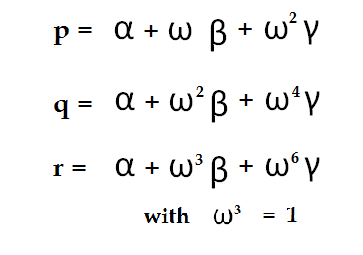Now, in general, the cube root of unity is not an element of the splitting field of our cubic. So the three expressions I've listed are outside the splitting field. Except for the third one, that is...it obviously reduces to the sum of alpha, beta, and gamma, which is just a rational number. In fact it's the coefficient of the x-squared term in our cubic, which we can easily force to zero by a fairly simple linear shift.

Notice that if we add these three expressions together, the betas and gammas all go away, and we're left with alpha. That's the root we're looking for. Now we're about to show how we get it. The three expressions I've written are all the cube roots of things we can evaluate! The third one (r) trivially so, since it's the cube root of zero, or at worst the cube root of an ordinary number. But try cubing either p or q. You get almost the same result, whichever one you cube. You get terms in alpha-cubed, beta-cubed, and gamma-cubed. Because those are symmetric in alpha, beta, and gamma, they evaluate out to ordinary rational numbers. You get terms in alpha-beta-gamma....again, these are symmetric terms which are easily evaluated as simple multiples of the zero-order coefficient of the cubic. And then...then, you get terms in alpha-squared beta and beta-squared gamma. These terms are mixed in with the omegas....but when you work them out, you can group them as a term expressible as the symmetric sum of alpha-squared betas etc....hence a rational number....and a term which is the difference  of alpha-squared betas take-away beta-squared alphas etcetera. This is the w expression I talked about the other day. It's the square root of an ordinary number, and that number is easily evaluated by writing it out in terms of the elementary symmetric polynomials.

In other words, p and q are just the cube roots of an ordinary number plus the square root of an ordinary number. And I wrote this out explicitly a couple of posts ago: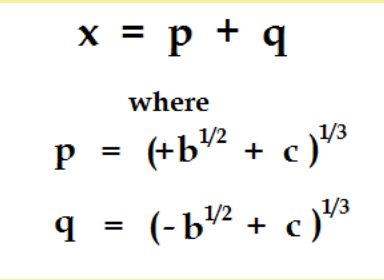At that time I simply declared that the solution of the cubic had this form. Now I'm showing you why it works. By working out their algebraic structure, I've identified our p's and q's with those funny combinations of alpha, beta and gamma mixed together with our cube roots of unity. We can see that they have this structure by cubing them out and verifying directly that the pieces making them up are nothing more than ordinary numbers and the square roots of ordinary numbers.

And that's how you solve the cubic equation. Anyone want to see how the fourth degree works out?

## Friday, June 22, 2012

### The Fifth Degree: Part IV

I've been reading over what I posted yesterday about the cubic, where I identified a certain combination of the roots alpha, beta and gamma as the square root of a rational number: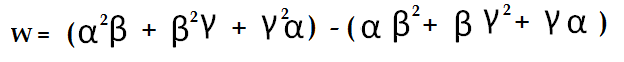Now I'm not all that happy with the way I explained this. All those terms in alpha-squared-beta, etc....those six functions really define everything about the structure of the algebraic field formed by adjoining alpha, beta, and gamma to the rationals. The field structure derives from the indistinguishability of the three roots. With respect to the rational numbers, the three roots are in general indistinguishable with respect to re-shuffling. This means that if two people evaluate any expression in alpha, beta and gamma, it doesn't matter if they make different choices for which one is which...the result, expressed in terms of rational numbers, will be the same either way.

Actually, this isn't always true. It's clearly not true for a cubic which factors, where one of the roots is rational and the others aren't. Even some irreducible cubics have roots that cannot always be shuffled indiscriminately. (These are cases where the roots have a cyclic relationship.) But in the most general case, the three roots can be freely interchanged with each other.

Let's look at the implications of this for our six terms in alpha-squared-beta, etc. Any re-shuffling of alpha, beta and gamma will result in a re-shuffling of our alpha-squared betas etc. For example, if we swap beta and gamma, then alpha-squared-beta becomes alpha-squared-gamma. But there is one interesting function in our alpha-squared-betas that has an interesting property. Consider: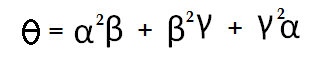If you start swapping alphas and betas with each other, you notice a peculiar thing. There are only two possible outcomes. Either you end up with theta unchanged, or you end up with what I'll call theta-prime: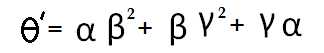Try it if you don't believe me. Any operation whereby you switch the alphas, betas and gammas with each other can only have one of two outcomes on the thetas: either they stay where they are, or they trade places with each other. And that means our thetas are the roots of a quadratic equation.

Furthermore, it's easy to see that they are the roots of a quadratic. Just take their sum and their product. Our thetas are not quite symmetric functions in alpha, beta and gamma. They are just a bit unsymmetric. But their sum and product are both symmetric functions, and as such, must have rational values. (As I pointed out last time, this property is inherited from the elementary symmetric functions in alpha, beta and gamma, which are just the coefficients of the cubic equation.)

When I tried to explain this yesterday, I basically started from the position that the difference of the thetas is the square root of a rational. Well, that's true for the roots of any quadratic equation, but I don't think it was the best way to make my point...not when I get around to generalizing the argument to equations of higher degree.

What do we really gain by the existence of the thetas? The huge consequence, the thing that Galois recognized, is this: that we have the field of rational numbers, and we have the field of algebraic numbers formed by adjoining the roots of the cubic to the rationals: these are the fields that we already knew about. But now we have a third field, intermediate between these two fields, which is formed by adjoining an ordinary square root to the rationals. And this intermediate field is the stepping stone which helps us reach our ultimate destination, the field which is known as the "splitting field" of the cubic.

Now, I took a course in this stuff many decades ago, and I distinctly remember that we supposedly reach the splitting field by adjoining nth roots of an element of each successive field. So having reached this intermediate field, you'd think we simply adjoin the cube root of some element and this generates the destination field.

Incredibly, it doesn't seem to work that way. We are, for the sake of argument, sitting in the field formed by adjoining the square root of seven to the rationals. Now take a typical element of this field, such as twelve take-away four root seven, and cube root it. Adjoin this number to the field we already had: this gives us: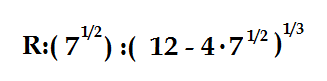where we've created this extension field first by adjoining the square root of an ordinary element of our starting field, the rationals; and then by adjoining the cube root of a typical element in the secondary field. And yet: this is not the splitting field of a cubic. We have to adjoin one more element: the conjugate of the last element we adjoined. It's not enough to tack on one without the other. We have to make both choices of the square root: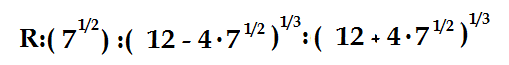And needless to say, it wasn't enough to adjoin just one of the cube roots: we have to adjoin all three cube roots of each of the two conjugates.

Is this new field the splitting field of our cubic? Actually, no. It's a bigger field than our splitting field. It includes elements that are not  part of the true splitting field, the field formed by the algebraic closure of alpha, beta, and gamma with the rationals. This field, the true splitting field, doesn't include our two conjugate cube root terms. Our alpha, beta and gamma are composed of the sums, suitably chosen, of those conjugate cube roots.

Furthermore, the whole ball of wax only works in the special circumstance that the product of our two conjugates happens to be a perfect cube. In the example I've chosen, we evaluate the product as 144 - 16*7 = 32....not a perfect cube. So this doesn't happen to be the splitting field of any cubic equation with rational coefficients.

It's all very peculiar.

## Tuesday, June 19, 2012

### The Fifth Degree: Part III

When we left off, I had shown an expression in rational numbers b and c which I claimed gave us three unique values for the solutions of a cubic equation. This would be a small miracle because by the time you've taken square roots of this and cube roots of that, you have at least six possible values for the simplest possible expression. In fact, the expression I gave ought to have had nine possible values: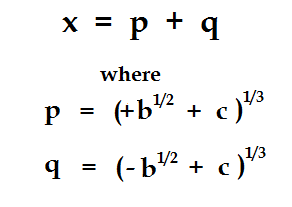You choose one of three values from column A, and one of three values from column B. Nine values for x. How is that the solution of a cubic?

It's not. It's the solution of a ninth-degree equation. But...and here's the catch...sometimes, the ninth degree reduces to a cubic. If c-squared take-away b happens to be a perfect cube....look what happens. For example, if c is 5 and b is -2, then the expression pq, as usual, takes on nine possible values. But three of those values come out to pq = 3. (The other six values are equal to 3w, where w is a cube root of unity.) What it means is that while all three p's are indistinguishable from each other, and all three q's are also indistinguishable, not all three pairs of p and q are indistinguishable. We choose only those pairs p and q whose product is a rational number. And those pairs give us three unique values which are the solution of a cubic equation.

It's fun to work it back from the other direction. Let the three solutions of our cubic equation be alpha, beta, and gamma. It's not too hard to show that linear combinations of all polynomials in alpha, beta, and gamma up to second degree in any variable form an algebraically closed field. (Obviously you don't have to consider third powers of alpha etc. because these always reduce to expressions in lower powers using the cubic equation itself. In particular, we can identify one particular element of this algebraic field which we will see is the solution of a quadratic equation. Take a deep breath, because here goes:(CORRECTION: When I first posted this, I inadvertently squared one of the bracketed terms.) In fact, w is not just the solution of a quadratic equation, its the square root of a rational number. There are two ways we can see this. First, we can square it out and examine the terms. But we don't have to work quite that hard. Remember, every symmetric polynomial in alpha, beta, and gamma is a rational numbers. This property is inherited from the elementary symmetric polynomails: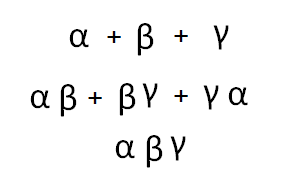You should recognize these as the rational coefficients of the cubic equation. So they are all just numbers. And it's not hard to show that any symmetric combination of alpha, beta, and gamma can be expressed as a function of these three elementary building blocks. So if it's symmetric, it's a rational number.

Now, the expression we built for w is not quite symmetric, but it's not far from it. If we take the square of w, we get six symmetric terms in alpha-squared-beta etc, and then we get a bunch of cross terms which, when the dust has all settled, turn out to be symmetric as well. So w-squared is rational.

There's another way we could have seen this by looking at the group theoretic  properties of the field. For a general cubic equation, you can take any permutation of the three roots and create a new field identical to the original field, but with the numbers simply shuffled around. Except for one thing: you can't shuffle around the rational numbers. Everything else is fair game. It's the logical consequence of alpha, beta and gamma being indistinuishable from one another. If I swap alpha and beta, everything I do with them algebraically has to have the same ultimate result at the point where I write a formula that evaluates to a rational value. I'm not allowed to complain that this irrational number is different from that irrational number, because the only properties that really count are those that lead to rational numbers. So I can swap alpha and beta, leaving gamma fixed. I can shift alpha to beta, beta to alpha, and alpha to gamma. The irrational numbers all shuffle themselves accordingly, but the rationals stay fixed.

But what about our w, the expression I said was a square root? If you look at it carefully, you should see that if we switch alpha and beta, it simply reverses the sign of w. If we switch beta and gamma....same thing. If we cycle alpha to beta, beta to gamma, and gamma to alpha....we just get w itself, without even a change of sign.

What kind of number is algebraically indistinguishalbe from its own negative? Why, the square root of a number. The positive square root of two is indistinguishable from the negative square root of two. The field which you get by adjoining the square root of two to the rationals is a field with only one homomorphism of itself...the homomophism generated by swapping the positive and negative square roots of 2. If our w has this property, then it must be the square root of a rational number.

It is the existence of w that makes the cubic equation solvable. I think we'll talk about this tomorrow.

## Monday, June 18, 2012

### The Fifth Degree: Part II

When we left off, I said there was a problem with the solution of the cubic equation. Any formula powerful enough to do the job would generate too many solutions. Once you take the square root of this and the cube root of that, you already have six values. How do you write a formula that only generates three values, as required?

The solution to this problem is beautiful and unexpected. First let's look again at the hypothetical form I had for a solution of the cubic: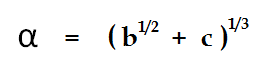This is actually a slight simplification. You may remember that I had three terms: a,b, and c in my earlier formula. I've thrown out the "a" term because it represents a simple linear shift that's easy to get rid of by simple algebra no matter what the degree of your equation. The formula I'm showing here is the basic form, and like I said, it generates six values instead of the required three.

Here's the funny thing: we can list all six values, and it turns out they can be combined with each other in a particular way that reduces them to just three values. We start by noticing that there are two groups of values for the cube roots, depending on our choice of the square root. For alternate values of the square root of b, we can call our cube roots p and q: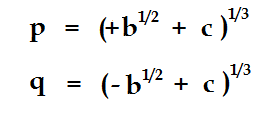You shouldn't think of the positive and negative square roots of b as separate and identifiable numbers. They are indeed two different numbers, but there is at the same time something indistinguishable about them. If b is a negative number, then they represent alternate choices for the imaginary i. It should be obvious that the sign of i is a simple matter of human convention, and that mathematically speaking there is no way to distinguish the "positive" and "negative" square roots of minus one. What is not so obvious to most people is that in terms of algebra, the positive and negative square roots of ordinary numbers like 2 are just as indistinguishable! At first thought, this is crazy talk...the number 1.414... is clearly a different number from the number -1.414...: one is positive and one is negative. This is not the right way to think about it! In algebra, a number is defined strictly by its algebraic properties with respect to the rational numbers. All we can say about the number we call "the square root of two" is that when you square it, you get the rational number 2. It is simply outside the scope of the discussion to say that one is positive and the other is negative.

The same goes for the cube roots! We know there are three cube roots of two: the real one, and the two complex ones located 120 degrees apart on the circle passing through the real root. Here is the strange thing about algebra: there is no meaningful distinction between those three roots! You can't tell them apart algebraically. What's more, it does you no good to try and tell them apart.

Of course, this doesn't apply to the cube roots of eight. The real cube root is 2, a rational number, and the complex roots are different numbers altogether. The two complex roots are indistinguishable from each other, but the rational root is special.

What about our p's and q's....where p and q are distinguished by the choice of the square root? Here it gets a little more interesting. Let's start by listing the six values for the expression. Letting omega be a complex cube root of unity (it could be either one, it doesn't matter!) we get these six terms: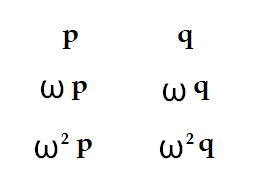I pointed out last time that these six terms are actually the roots of a sixth-degree equation. Now comes the magical part. By mixing and matching, I can construct the roots of a cubic equation. First I'm going to tell you what they look like, and then we'll talk about why it works. First, here is what the roots look like: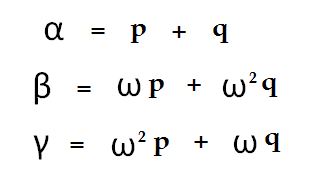We've taken six expressions and collapsed them down to three expressions. Two choices for the square roots and three choices for the cube root, and yet in the end we get three unique values.

Or do we? Haven't I cheated? Earlier I claimed that the three cube roots of a number are algebraically indistinguishable from each other. If they really are, then how am I entitled put the three cube roots in a specific order? In particular, I choose "p+q" as my alpha, from which beta and gamma follow. What would have stopped me from choosing , for example: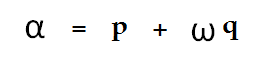as my first root....then I would have gotten entirely differnt values for beta and gamma as well.  For that matter, I might have chosen: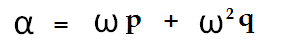but that wouldn't have hurt me, because while I would have gotten correspondingly different values for alpha, beta and gamma, they would have been the same three values that I got the first time around....just their names would have been swapped. But the other choice....where I take p+wq....that gives me three different values altogether. If I really can't distinguish between the three cube roots of a number, how do I choose which cube roots line up with each other? Do I have to take recourse to Cartesian geometry and recognize the "real" cube root as being distinguished from the others? Or is there some purely algebraic property which lets me identify three unique values for the roots of the equation?

It's a funny question and I think I'll leave the answer for tomorrow.

## Sunday, June 17, 2012

### The Fifth Degree: Part 1

I'm kind of stalled on physics these days, so maybe this would be a good time to catch up on some math topics. Let's see where this one goes...

One of the most mind-boggling acheivements in the history of human thought is the proof that the fifth degree equation is unsolvable. It's one thing to solve a hard equation, and another thing to prove that you can't solve it. It's a fascinating story that isn't well told.

We torture high school students with the solution of the quadratic equation. Most people will remember it as the hardest formula they ever had to memorize. This completely misses the essential point about the solving the quadratic: the equation is the simplest possible equation that makes sense. Reduced to its essence, the quadratic formula basically says: the solution of the quadratic equations is a number plus the square root of a number: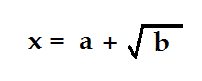It's a flawless formula because the number b has two square roots, and there are two solutions of the quadratic formula. Calling them alpha and beta, we can write: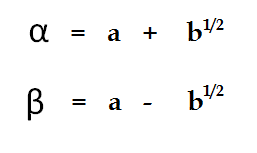The real beauty of the formula is that it doesn't ask you to distinguish between the positive and negative square roots. They are both correct, and they both give you solutions to the same equation. Incidentally, it's amazing how easy it is to solve a quadratic equation using this reasoning as compared to the univerally-taught method of "completing the square" that you remember from high school. You just have to remember understand that the coefficients of the quadratic equation are actually the sum and product of the roots: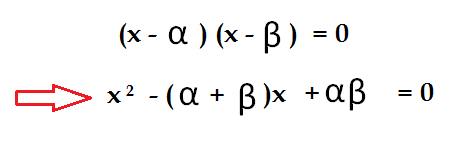The sum and product of alpha and beta are called the elementary symmetric polynomials in alpha and beta, and they have very simple expressions in terms of our a and b, those as-yet-undetermined numbers in which the roots will ultimately be expressed: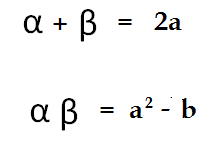Do you see that if we simply identify these last two expressions with the coefficients of the quadratic as expanded in the previous equation, that the quadratic solves amost effortlessly?

A fascinating thing happens when we move on to the cubic equation. Here again, the standard treatments are horribly misguided, by my way of thinking. Normally they take you through some very difficult algebra whose end result is a horribly intimidating mass of symbols. "This", they tell you, "is the solution of the cubic equation. The fourth degree is even more complicated."

Instead of emphasizing the complexity of the solutions, the correct approach is to recognize their simplicity. We look for the simplest possible solution of the cubic equation: and by analogy with our simplified solution to the quadratic equation, we look first to the obvious: a number plus the cube root of a number: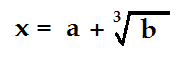This is our trial solution, and a few moments reflection is sufficient to show it doesn't do the job. It starts of well: a cubic equation must have three solutions, and since the number b has three cube roots...so far, so good. Taking omega as a cube root of unity, we can even write the three roots out explicitly, as we did with the quadratic: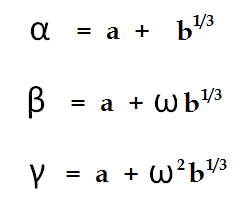It's a nice formula that takes on exactly three values...note that it doesn't matter if we take b to be a real or complex cube root, we get the same three values for alpha beta and gamma no matter what choice we make. But is soon becomes apparent that this simple formula just doesn't have enough versatility to take care of all the things a cubic equation can do. For one thing, it obviously can't generate three real values, which rules out all kinds of perfectly good equations. We need something more complicated.

In particular, since every quadratic equation is also a cubic equation (albeit with a leading coefficient of zero), we need a formula that allows for taking square roots as well as cube roots. The least complicated formulas that fill these minimal requirements would be something like this: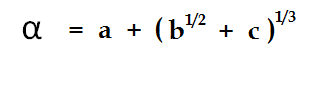Or perhaps something similar with the cube root inside the brackets instead of the square root. Either way, we are at least making progress. But there is something horribly wrong with this proposed solution. Where our previous guess was not powerful enough to do the job, this one is too powerful. Or at least, it generates too many solutions. With our quadratic equation, we had a formula that generated two solutions, which was just what we needed. Now we have a formula that generates six solutions: two choices for the square root, and three choices for the cube root. That's twice as many solutions as we want. It's not a good sign.

In fact, it's not too hard to verify that formula we've written is the solution of a fairly simple sixth-degree equation in alpha. You can generate the exact equation by cubing both sides, collecting terms, and then squaring both sides again. But that's not where we want to go with this.

The real question is: how do we write a solution for the cubic equation that is powerful enough to do the job, and at the same time doesn't generate too many solutions?

Let's take that up when we return.